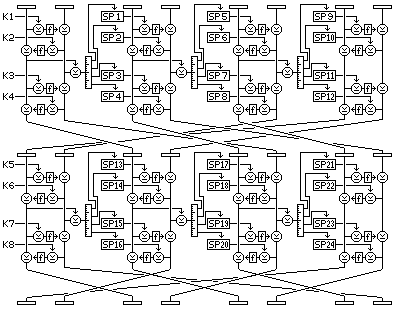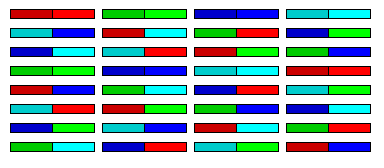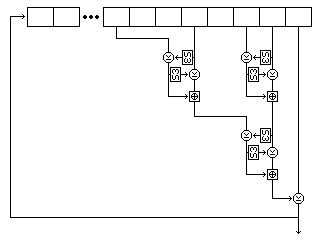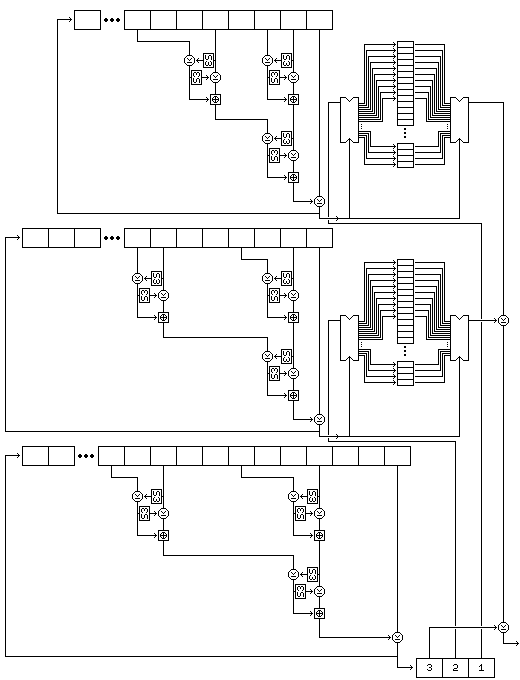[Next] [Up] [Previous] [Index]

Quadibloc VII is an attempt to embody the principles found in the Large-Key Brainstorm within the compass of a block cipher.

The subkey material it uses consists of:

• Two S-boxes, each containing 256 entries, each entry being 16 bits in length; (1024 bytes)
• Thirty-two subkeys, four for each of eight rounds, each 16 bits in length; (64 bytes)
• Ninety-six subkey pools, each one containing 16 subkeys, each subkey being 16 bits in length, (3072 bytes)

for a total of 4,160 bytes of subkey material.

### The Rounds

The first two rounds of Quadibloc VII look like this:In Quadibloc VII, the 128-bit block is divided into four quarters, of 32 bits each, each of which is further divided into two 16-bit halves. Each round of Quadibloc VII consists of four Feistel rounds performed on each of these pairs of 16-bit halves.

The XOR of the two halves of the first 32-bit quarter after two Feistel rounds is used to control, for each of the four Feistel rounds performed on the next quarter, which of sixteen possible subkeys are used.

After every odd-numbered round, the eight 16-bit subblocks are permuted to the following order (expressed in terms of a list of the sources of the subblocks after the permutation):

```7 6 1 8 3 2 5 4
```

thus, the left halves move to the next later quarter, and the right halves move to the corresponding position in the other half of the entire block.

After every even-numbered round except the last, the eight 16-bit subblocks are permuted to the following order (expressed in terms of a list of the sources of the subblocks after the permutation):

```7 4 1 6 3 8 5 2
```

thus, the left halves move to the next later quarter, and the right halves move to the next earlier quarter.

This diagram illustrates, by color-coding, how the pieces of the block move during the 8 rounds of Quadibloc VII:and here is a table showing this in text form:

``` (1)    3   4    5   6    7   8
7   6   (1)  8    3     5   4
5   8    7    (1)  4    3   6
3   4    5   6    7   8   (1) 
(1)  6    3   8    5     7   4
7   2   (1)  4    3   6    5   8
5   4    7   6   (1)  8    3  
3   8    5     7   4   (1)  6
```

The paths of the first left half and the first right half are indicated by brackets. Note that the first left half, 1, is enciphered:

```with right half     in rounds
2            1, 4
4            3, 6
6            5, 8
8            7, 2
```

thus ensuring that the blocks affect the other blocks by being enciphered with them in the small Feistel rounds, in addition to affecting them by modifying their encipherment through the use of the subkey pools.

The f-function is merely the XOR of the value in S10 indexed by the leftmost half of the input with the value in S11 indexed by the rightmost half of the input.

### The Key Schedule

While the round structure of Quadibloc VII is impressive, as is to be expected given the large amount of subkey material it consumes, as there are only two S-boxes in the cipher, both of them key-dependent, the cipher is still only as good as its key schedule.

Initially, the subkeys will be filled in the following order: first the 96 subkey pools, then the two S-boxes (first S10, then S11, from entry 0 to entry 255 each), then the 32 fixed subkeys. And they will be initially filled by means of almost the same key generation method as used in Quadibloc S:

The key consists of two or more bytes.

The key is expanded to prevent a key that is long and of all zeroes in whole or in part from causing poor results as follows: a key of n bytes is expanded to one of 3n+1 bytes, the last byte of which is a byte equal to the inverse, the bitwise negation, or one's complement, of the XOR of all the bytes of the original n byte key. The first 3n bytes of the key alternate between a byte from each of the following sources:

• The n bytes of the original key, in order.
• One of the possible byte values, starting from 127, and incrementing by one each time.
• The bytes of the original key, in reverse order, inverted, and with 1, 2, 3, and so on added to them.

Thus, if the original key is 0 128 255, after expansion the key becomes 0 127 1 128 128 129 255 129 2 128.

Bytes are then generated from the key by chain addition. This means that a byte is generated as follows: the sum, modulo 256, of the first two bytes of the key is the generated result; and it is also appended to the end of the key, whose first byte is then removed. (Note that the cipher itself uses XOR only, and not addition modulo 256.)

The method of producing subkey bytes is a degenerate form of the MacLaren-Marsaglia generator. An array with 256 byte positions, called A(0) to A(255), is filled by generating 256 bytes by means of chain addition.

Then, a subkey byte is generated as follows:

Generate two bytes by chain addition. Call these bytes p and q.

The byte to be used in a subkey is the current value of A(q).

Replace A(q) with p.

Once all the subkeys have been filled by this method, the quantity 01F253A435C607F859AA3BCC0DFE5FA0 is to be enciphered with the temporary subkeys thus calculated, for the first four rounds of a normal Quadibloc VII encipherment.

This output is now used as the key from which bytes are generated by chain addition. It is expanded, but not in the same fashion as the original key: it is only doubled in length, and the bytes of the key alternate with the bytes of the key in reverse order, inverted (but without anything added to them). Since 32 is not a number of the form 3n+1 (unlike 16, which is such a number), both keys are ensured to be different in length.

Then, the degenerate MacLaren-Marsaglia procedure is to be repeated, with the bytes produced by it XORed with the subkey bytes in order.

### Revised Key Generation

The method used for generating subkeys for Quadibloc XI may be applied to this cipher as well. Here, for simplicity and to avoid confusion, S3 will be used as it was in Quadibloc XI (although the fixed S-boxes S1 and S2 are also potentially available). The key material is generated in the same order, the 96 subkey pools each containing sixteen 32-bit subkeys, the contents of S10 and S11, each with 256 sixteen-bit entries, and then the 32 regular subkeys.

For this method of subkey generation, the key must be a multiple of four bytes in length.

### Initialization

Three strings of bytes of different length are produced from the key.

The first string consists of the key, followed by one byte containing the one's complement of the XOR of all the bytes of the key.

The second string consists of the one's complements of the bytes of the key in reverse order, with three bytes appended containing the following three quantities:

• The sum, modulo 255, of the bytes of the key, incremented by one by normal addition. (Thus, this produces a number from 1 to 255.)
• The XOR of all the bytes at odd numbered positions in the key, where the first byte in the key is considered to be byte 1, and odd.
• The one's complement of the XOR of all the bytes at even numbered positions in the key.

The third string consists of alternating bytes, taken from the bytes of the key in reverse order, and then from the bytes of the one's complement of the key, and then that string is followed by the one's complements of the first four bytes of the key.

Thus, if the key is:

``` 128  64  32  16   8   4   2   1   1   2   3   4   5   6   7   8
```

then the strings generated from it are as follows:

```First string:
128  64  32  16   8   4   2   1
1   2   3   4   5   6   7   8
8

Second string:
247 248 249 250 251 252 253 254
254 253 251 247 239 223 191 127
37 170  93

Third string:
8 127   7 191   6 223   5 239
4 247   3 251   2 253   1 254
1 254   2 253   4 252   8 251
16 250  32 249  64 248 128 247
127 191 223 239
```

Given that the length of the key is 4n, the lengths of the three strings are 4n+1, 4n+3, and 8n+4, and hence all three are relatively prime, since both 4n+1 and 4n+3 are odd, and 8n+4 is two times 4n+2.

Two buffers, each containing room for 256 bytes, are filled by generating bytes from the first and second strings by placing them in a nonlinear shift register.

The form of that shift register is shown in the following illustration, showing its precise form for the first string.Five bytes are read from the string at each step. For the first string, they are, as shown in the diagram, the eighth-last, fifth-last, third-last, and second-last bytes and the last byte. For the second string, they are the eighth-last, seventh-last, fourth-last, and second-last bytes, and the last byte. For the third string, they are the twelfth-last, tenth-last, seventh-last, and fourth-last bytes, and the last byte.

Each time the shift register produces a byte, it does so as follows:

• The second byte read is used to select an entry in S-box S3, and the value of this entry is XORed to the first byte. Then the first byte is used to select an entry in S-box S3, and the value of this entry is XORed to the second byte.
• The fourth byte read is used to select an entry in S-box S3, and the value of this entry is XORed to the third byte. Then the third byte is used to select an entry in S-box S3, and the value of this entry is XORed to the fourth byte.
• The first and second bytes, as modified, are added together using modulo-256 addition to form a first result.
• The third and fourth bytes, as modified, are added together using modulo 256 addition to form a second result.
• The second result is used to select an entry in S-box S3, and the value of this entry is XORed to the first result. Then the first result is used to select an entry in S-box S3, and the value of this entry is XORed to the second result.
• The two results as modified are added together using modulo 256 addition to form a third result.
• The fifth byte read, which is always the last byte in the shift register, is XORed with the third result. The resulting value is output as the byte produced by operating the shift register.
• The values in the shift register are modified by removing the last byte, advancing the bytes in the shift register to the next later position, and appending the output result as the new first byte of the shift register contents.

Both buffers contain 256 bytes. The first buffer, called buffer A, is filled with 256 successive bytes generated from the second string by means of the nonlinear shift register filled with the second string. The second buffer, called buffer B, is filled with 256 successive bytes generated from the first string by means of the nonlinear shift register filled with the first string.

### Subkey Byte Generation

Once the setup is complete, subkey material is generated one byte at a time, the first byte generated being the leftmost byte of subkey K1, and so on.

A subkey byte is generated as follows:

• A byte is generated from the first string by the nonlinear shift register operation.
• The byte at the position in buffer A indicated by this value is taken, and called P.
• A byte is generated from the third string by the nonlinear shift register operation. In the case of the third string, the shift register operation involves reading the following five bytes: the twelfth, tenth, seventh, and fourth last bytes, and the last byte. The value of the byte thus produced is placed in buffer A, replacing the value taken.
• A byte is generated from the second string by the nonlinear shift register operation.
• The byte at the position in buffer B indicated by this value is taken, and called Q.
• A byte is generated from the third string by the nonlinear shift register operation. Its value is placed in buffer B, replacing the value taken.
• The subkey byte generated is the XOR of P and Q and an additional byte generated from the third string by the nonlinear shift register operation. (The use of an additional byte from the third string ensures that all the bytes of the key particpate directly in the key schedule; otherwise, some could be skipped over in a sense by the selection of bytes to use from the buffers.)

The following diagram attempts to illustrate the complete process of subkey byte generation:Note that this procedure, since it exercises the two strings used to select bytes, rather than the string used to generate values, results in a small change in the key resulting in large changes in the subkeys from the very beginning.

[Next] [Up] [Previous] [Index]